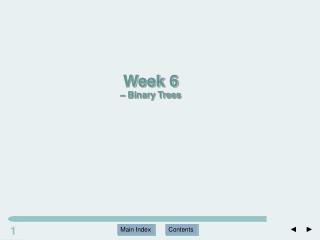# Main Index - PowerPoint PPT PresentationDownload PresentationMain Index

Main IndexDownload Presentation## Main Index

- - - - - - - - - - - - - - - - - - - - - - - - - - - E N D - - - - - - - - - - - - - - - - - - - - - - - - - - -
##### Presentation Transcript

1. 1 Main Index Contents Week 6 – Binary Trees

2. Sequence and Associative Containers • Sequence containers access data by position • Array (index) • Vector (index) • List (iterator) • Associate containers can access data by value • Set and Map / Binary search tree Arrays Vectors Linked lists Trees

3. Tree in Nature

4. Tree in our life • Need to turn it upside down

5. Tree in Computer Science • Similar to tree in nature Root Leaves

6. 6 Main Index Contents Tree Structures Root Parent Child Edge Leaf Interior node Subtree Level Depth = max level

7. Terminologies used in Trees - Wiki

8. 8 Main Index Contents Tree Node Level and Path Length What is the Depth?

9. Binary Tree Definition • A binary tree T is a finite set of nodes with one of the following properties: • (a) T is a tree if the set of nodes is empty. (An empty tree is a tree, size=0.) • (b) The set consists of a root, R, and exactly two distinct binary trees, the left subtree TL and the right subtree TR. The nodes in T consist of node R and all the nodes in TL and TR. • Any node in a binary tree has at most two children

10. 10 Main Index Contents Selected Samples of Binary Trees Does tree B have left subtree TL?

11. Density of a Binary Tree • Intuitively, density is a measure of the size of a tree (number of nodes) relative to the depth of the tree. • Trees with a higher density are important as data structures, because they can “pack” more nodes near the root. • Access to the nodes is along relatively short paths from the root.

12. Complete binary tree

13. Degenerate tree • A degenerate (or pathological) tree is where each parent node has only one associated child node. This means that performance-wise, the tree will behave like a linked list data structure.

14. Evaluating Tree Density • Complete binary trees are an ideal storage structure, because of their ability to pack a large number of nodes near the root • Assume we want to store n elements in a complete binary tree. We would like to know the depthd of such a tree.

15. Depth d--- Size n in complete binary tree 20 = 1 nodes 21 = 2 nodes Geometric series level d has 2d nodes … … … ………………… -1

16. 17 Main Index Contents Binary Tree Nodes

17. Node class template <typename T> { public: T nodeValue; tnode<T> *left, *right; tnode() {} tnode(const T& item, tnode<T> *lptr=NULL, tnode<T> *rptr=NULL):nodeValue(item), left(lptr), right(rptr) {} };

18. Node structure

19. Building a Binary Tree • A binary tree consists of a collection of dynamically allocated tnode objects whose pointer values specify links to their children.

20. Recursion • Solution to a problem depends on solutions to smaller instances of the same problem. • As a tree is a self-referential (recursively defined) data structure, traversal can naturally be described by recursion. • Recursive function: a function that calls itself.

21. BINARY TREE SCAN ALGORITHMS • How to traverse the tree so that each node is visited exactly once? 1. Depth-first • Pre-order • In-order • Post-order 2. Breadth-first (level-order)

22. Depth-first • Defined as operations recursively at each node. • The actions include: • visiting the node and performing some task (N), • making a recursive descent to the left subtree (L), • making a recursive descent to the right subtree (R). • The different scanning strategies depend on the order in which we perform the tasks.

23. In-order Scan • The prefix “in” comes from the fact that the visit occurs between the descents into the two subtrees. • In this example, we use the order LNR. 1. Traverse the left subtree (“go left”). 2. Visit the node. 3. Traverse the right subtree (“go right”). N R L Recursively!

24. In-order example L N R   L N R L N R L N R N   R L Recursively! In-order scan: B, D, A, E, C

25. In-order output

26. Post-order scan • 1. Traverse the left subtree (“go left”). • 2. Traverse the right subtree (“go right”). • 3. Visit the node. D, B, E, C, A 3. 2. 1.

27. Post-order output

28. Pre-order scan 1. Visit the node. 2. Traverse the left subtree (“go left”). 3. Traverse the right subtree (“go right”). 1. A, B, D, C, E 3. 2.

29. Breadth-first (Level-Order) Scan

30. Example Question F B G A D I C E H Pre-order? In-order? Post-order? Level-order ?

31. WIKI

32. Computing the Leaf Count Pre-order scan

33. Computing the Depth of a Tree Post-order scan

34. Deleting Tree Nodes Post-order scan

35. Reading • Chapter 4